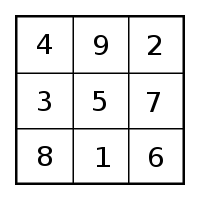# Creation: Phi & Exponential factor are in Genesis 1:1Creation: Phi & Exponential factor are in Genesis 1:1. בְּרֵאשִׁית, בָּרָא אֱלֹהִים, אֵת הַשָּׁמַיִם, וְאֵת הָאָרֶץ. Translated to English, “In the beginning God created the Heavens and the Earth.” First of all, please review my blog on the ““The Broadway Marquee of Megalithic Measure“. The picture below is the “Broadway Marquee” of antiquity referred to. That particular blog shows a few of its hidden codes. Here is a brief review:Creation: This is the Broadway Marquee of Megalithic Measure
• In Greek and Middle eastern languages letters doubled as numbers. Therefore, every letter, word, phrase, sentence, etc. had a numerical import.
• The 1st sentence of Genesis equals in Hebrew is 2701. One was often added by traditional of the Colel. I think “colel” represented the singular Deity, John Michell discusses the subject more thoroughly in his numerous works.   Work with your calculator to double check me. Add the numbers around the central 5. The eight numbers total 40. Then add them two at the time overlapping the numbers as 49 + 92 + 27 + 76 + 61 + 18 + 83 + 34 = 440. Now take the number three at the time. Overlap the third with the first number as: 492 + 276 + 618 +  834 = 2,220. They come to the same totals in reverse. Let (P) stand for perimeter. Then (p-1)   is 40 + (P-2)  is 440 + ( P-3) is 2,220  = 2700. Add one for the Deity and you have 2701.This number square defines the perimeters of Creation.

### What Else Does the 1st sentence of Genesis Have to Say About Creation?

Those who know Hebrew can, and I’m sure will, double check my figures. To the best of my knowledge, they are accurate. Anyone can see the 1st sentence has seven words. Hebrew is read from left to right. The 1st letter of each of the first five words factor phi. That is the ratio by which nature unfolds by. If you never hear of it, check it out on line.

The number that nature loves to work with is called in Greek by the name “phi.”  The Greek letter phi ($\varphi$ or$\phi$) represents the golden ratio. Its value is:$\varphi = \frac{1+\sqrt{5}}{2} = 1.6180339887\ldots.$(this was copied from Wikipedia).

Here are the factors of phi as found in Genesis. Take the first 5 letters (left to right) to get the picture.  Beis is 2. The 1st two words of the 7 letter first sentence each start with a “beis” or number “2”.   Aleph is 1: The next two words start with an aleph. The fifth word starts with a “hei”, the symbol for 5. Now, 1,5, and 2. Immediately, we have all 3 factors of phi: 1,2, and 5.
The next mathematical “gem” of nature is the exponential factor of growth. This is also a key part of the math of creation. It places limits on how quickly system can grow. The factor is 2.72 (rounded figure).  Look at the first letters of the last two words. The 1st letter is a “vav” which is the symbol for 6. The last letter is a “hei” which is the symbol for 5. The formula for the exponential factor is 6 X 5/6+5 or 30/11 = 2.727272……..
Pi is the classic circle and diameter as pictured below. The 7 words with “Elohim” representing the center becomes the 3rd mathematical gem. It also represents the central Sabbath surrounded by the other 6 days of the week. There is so much to blog about just on the 1st sentence of Genesis. More will appear, Keep watching.Creation follows an orderly model or pattern

## 1 Comment

1.michael kors handbags outlet says:

Your home is valueble for me. Thanks!?